MORE IN Analog Electronics
Total marks: --
Total time: --
INSTRUCTIONS
(1) Assume appropriate data and state your reasons
(2) Marks are given to the right of every question
(3) Draw neat diagrams wherever necessary

1 (a) Explain the following with respect to semi conductor diode:
i) Diffusion capacitance
ii) Transition capacitance
iii) Reverse recovery time.
6 M
1 (b) Explain the working of bridge rectifier.
8 M
1 (c) Design a suitable circuit represented by the box shown below which has input and output waveforms as indicated.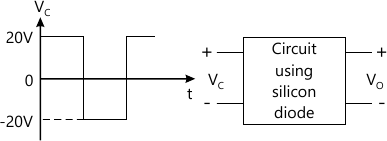Q.1(C)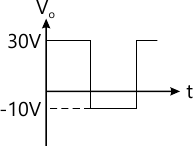Q.1 (C)(ii)

6 M

2 (a) Derive an expression for IB, IC and VCE for voltage divider bias using exact analysis.
10 M
2 (b) For the circuit shown below determine (i) Ia (ii) IC (iii) VCE.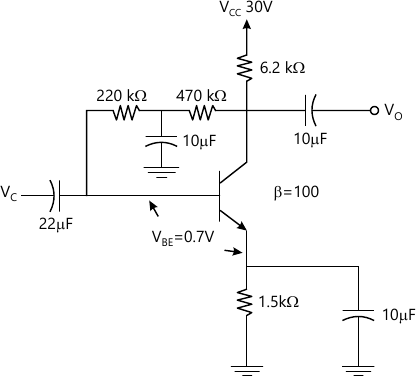2(b)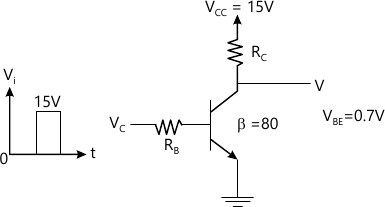2(b)(ii)

6 M
2 (c) Determine RB and RC for the transistor inverter of Fig. Q2(c) if IC(sat)=10 mA. IB=150 IB|max|.
4 M

3 (a) Derive an expression for Av, Z1 and Zo for CE fixed bias using rc equivalent model.
10 M
3 (b) For the circuit shown in Fig. Q3 (b), calculate re, Z1, ZO, AV, AI.

B=200, VCC=0.7V, ro=60kΩ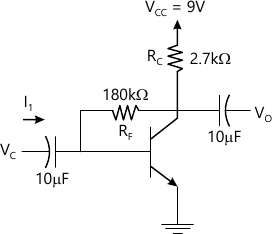10 M

4 (a) Determine the lower cut off frequency for the emitter follower using BJT amplifier with Cs=0.1 μf. Rs=1 KΩ. R1=120 KΩ. R1=4KΩ. RF=1.5 KΩ. CC=0.1 μf. β=100 ro&infty;, VCC=15V. VBF=0.7V.
12 M
4 (b) Derive equation for Miller input and output capacitance.
8 M

5 (a) Derive an expression for Zi, Av, At for Darlington emitter follower circuit.
10 M
5 (b) What are the effects of negative feedback in amplifier? Show how bandwidth of an amplifier increase with negative feedback.
10 M

6 (a) With a neat diagram explain the working of complementary symmetry push pull amplifier.
10 M
6 (b) Explain the operation of class B push pull amplifier and show that its efficiency is 78.5% at maximum power dissipation.
10 M

7 (a) With a neat diagram explain the working of RC phase shift oscillator.
8 M
7 (b) With a neat diagram explain the working of series resonant crystal oscillator. A crystal has L=0.334 H, C=0.065 pf, CM=1 pf, R=5.5 kΩ. Calculate its series and parallel resonating frequency.
12 M

8 (a) Draw the JEET amplifier using fixed bias configuration. Derive Zi, ZO and AV using small signal model.
10 M
8 (b) For the JFET amplifier shown in Fig. Q3(b). calculate (i) gm (ii) rd (iii) Zi (iv) Zn (v) Av
IPSS=5mA
VP=-6V
YOS=40 μs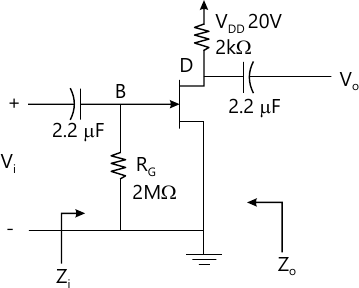10 M

More question papers from Analog Electronics